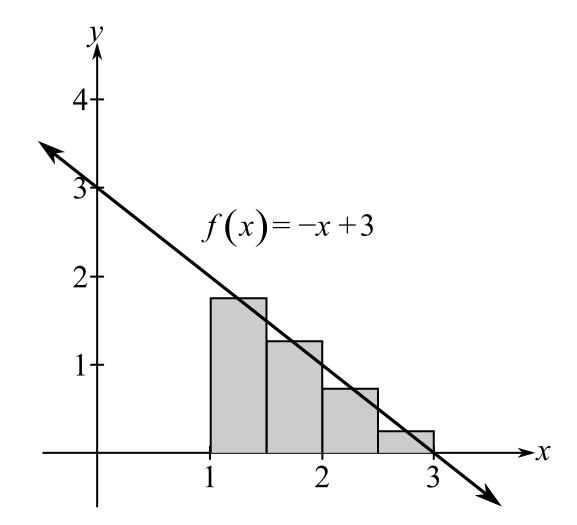Chapter 5.6, Problem 1E### Calculus: An Applied Approach (Min...

10th Edition
Ron Larson
ISBN: 9781305860919

#### Solutions

Chapter
Section### Calculus: An Applied Approach (Min...

10th Edition
Ron Larson
ISBN: 9781305860919
Textbook Problem
1 views

# Approximating the Area of a Plane Region In Exercises 1-6, use the rectangles to approximate the area of the region. Compare your result with the exact area obtained using a definite integral. See Example 1. f ( x ) = − x + 3 , [ 1 , 3 ]To determine

To calculate: The area bounded by the function f(x)=x+3 between interval [1,3] by mid-point rule. Also compare this approximate area with exact area using definite integral.Explanation

Given Information:

The provided function is f(x)=x+3. Area of this function is calculated between the interval [1,3] as shown below:

Formula used:

The approximate area of any definite integral baf(x)dx by the use of midpoint rule is calculated by the use of following steps.

Step1: First divide the provided interval of the function into n sub intervals by the use of formula;

Δx=ban where [a,b] are the intervals and n is the subinterval value and Δx is the width.

Step2: Use above subinterval find the midpoint of each sub interval.

Step3: Final step is to obtain approximate area by calculating function f at each mid-point from the use of formula;

abf(x)dxban[f(x1)+f(x2)+f(x3)+....+f(xn)].

Calculation:

Consider the provided function f(x)=x+3. The graph of provided function is shown below:

In provided graph, interval [1,3] is divided into 4 rectangle.

Now, put n=4, a=1 and b=3 to find the width of each rectangle as;

Δx=314=0.5

Width Δx of each rectangle will be 0.5.

So, the interval [1,3] will be divided into 4 subintervals as;

[1,1.5],[1.5,2],[2,2.5],[2.5,3].

Now, the value of midpoint of each rectangle is calculated as;

[x1,x2]=x1+x22 where x1,x2 are the upper value and lower value of the sub-interval

So, for first rectangle [1,1.5] mid-point is;

1+1.52=1.25

Similarly, for second rectangle [1.5,2] mid-point is;

1.5+22=1.75

For third rectangle [2,2.5] mid-point is;

2+2.52=2.25

For last rectangle [2.5,3] mid-point is;

2

### Still sussing out bartleby?

Check out a sample textbook solution.

See a sample solution

#### The Solution to Your Study Problems

Bartleby provides explanations to thousands of textbook problems written by our experts, many with advanced degrees!

Get Started

#### Find more solutions based on key concepts